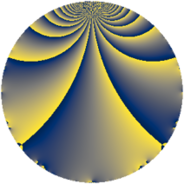# Properties

 Label 231.2.eLevel $231$ Weight $2$ Character orbit 231.e Rep. character $\chi_{231}(188,\cdot)$ Character field $\Q$ Dimension $28$ Newform subspaces $1$ Sturm bound $64$ Trace bound $0$

# Related objects

## Defining parameters

 Level: $$N$$ $$=$$ $$231 = 3 \cdot 7 \cdot 11$$ Weight: $$k$$ $$=$$ $$2$$ Character orbit: $$[\chi]$$ $$=$$ 231.e (of order $$2$$ and degree $$1$$) Character conductor: $$\operatorname{cond}(\chi)$$ $$=$$ $$21$$ Character field: $$\Q$$ Newform subspaces: $$1$$ Sturm bound: $$64$$ Trace bound: $$0$$

## Dimensions

The following table gives the dimensions of various subspaces of $$M_{2}(231, [\chi])$$.

Total New Old
Modular forms 36 28 8
Cusp forms 28 28 0
Eisenstein series 8 0 8

## Trace form

 $$28q - 32q^{4} - 8q^{7} - 8q^{9} + O(q^{10})$$ $$28q - 32q^{4} - 8q^{7} - 8q^{9} - 20q^{15} + 40q^{16} - 12q^{18} - 10q^{21} + 36q^{25} + 12q^{28} - 4q^{30} + 24q^{36} - 24q^{37} + 16q^{39} - 40q^{43} - 16q^{46} + 4q^{49} - 8q^{51} - 4q^{57} - 44q^{58} + 52q^{60} + 6q^{63} - 68q^{64} + 40q^{67} + 20q^{70} + 24q^{72} - 28q^{78} + 56q^{79} + 32q^{81} + 100q^{84} - 8q^{85} + 12q^{88} + 8q^{91} - 36q^{93} + O(q^{100})$$

## Decomposition of $$S_{2}^{\mathrm{new}}(231, [\chi])$$ into newform subspaces

Label Dim. $$A$$ Field CM Traces $q$-expansion
$$a_2$$ $$a_3$$ $$a_5$$ $$a_7$$
231.2.e.a $$28$$ $$1.845$$ None $$0$$ $$0$$ $$0$$ $$-8$$